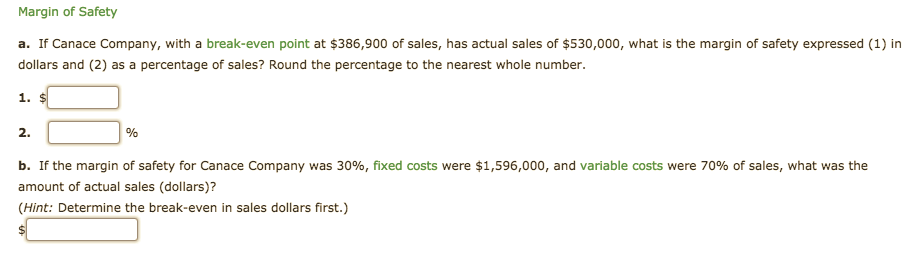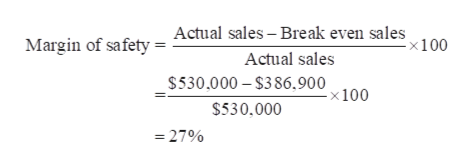# Margin of Safetya. If Canace Company, with a break-even point at \$386,900 of sales, has actual sales of \$530,000, what is the margin of safety expressed (1) indollars and (2) as a percentage of sales? Round the percentage to the nearest whole number.1. \$2.b. If the margin of safety for Canace Company was 30%, fixed costs were \$1,596,000, and variable costs were 70% of sales, what was theamount of actual sales (dollars)?(Hint: Determine the break-even in sales dollars first.)

Question
21 viewshelp_outlineImage TranscriptioncloseMargin of Safety a. If Canace Company, with a break-even point at \$386,900 of sales, has actual sales of \$530,000, what is the margin of safety expressed (1) in dollars and (2) as a percentage of sales? Round the percentage to the nearest whole number. 1. \$ 2. b. If the margin of safety for Canace Company was 30%, fixed costs were \$1,596,000, and variable costs were 70% of sales, what was the amount of actual sales (dollars)? (Hint: Determine the break-even in sales dollars first.) fullscreen
check_circle

Step 1

a. 1. Calculate the margin of safety (in Dollars).

Step 2

a. 2. Calculate the margin of safety (as a percentage of sales).help_outlineImage TranscriptioncloseActual sales - Break even sales -x100 Margin of safety Actual sales \$530,000 \$386,900 -x 100 \$530.000 27% = fullscreen
Step 3

Calculate the contribution marg...

### Want to see the full answer?

See Solution

#### Want to see this answer and more?

Solutions are written by subject experts who are available 24/7. Questions are typically answered within 1 hour.*

See Solution
*Response times may vary by subject and question.
Tagged in